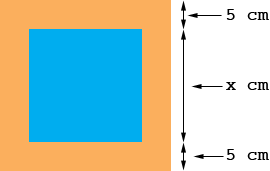To Whom It May Concern, I'm having some difficulty with these word problems. Maybe you could help. Thanks. A package designer wants to protect an expensive book with a 5 cm wide cardboard rim. The area of the rim is equal to the area of the book. What is the length of the book? (The book is square) Thanks for your help!! From, Karen Hi Karen, I immediately sketched a diagram to make sure that I understood the problem.The blue region is the book and the orange region is the rim. I know the rim is 5 cm wide but I don't know the height of the book so I called it x cm. The book is square so its width is also x cm and thus the area of the book is xx = x2 square cm. The height of the book with the rim attached is 5 + x + 5 = 10 + x cm, and the width of the book with the rim attached is also 10 + x cm so the area of the book with the rim attached is (10 + x)(10 + x) = (10 + x)2 square cm. The instructions say that the area of the rim is equal to the area of the book so the area of the book and rim combined is twice the area of the book. That is (10 + x)2 = 2 x2 Solve for x. Penny